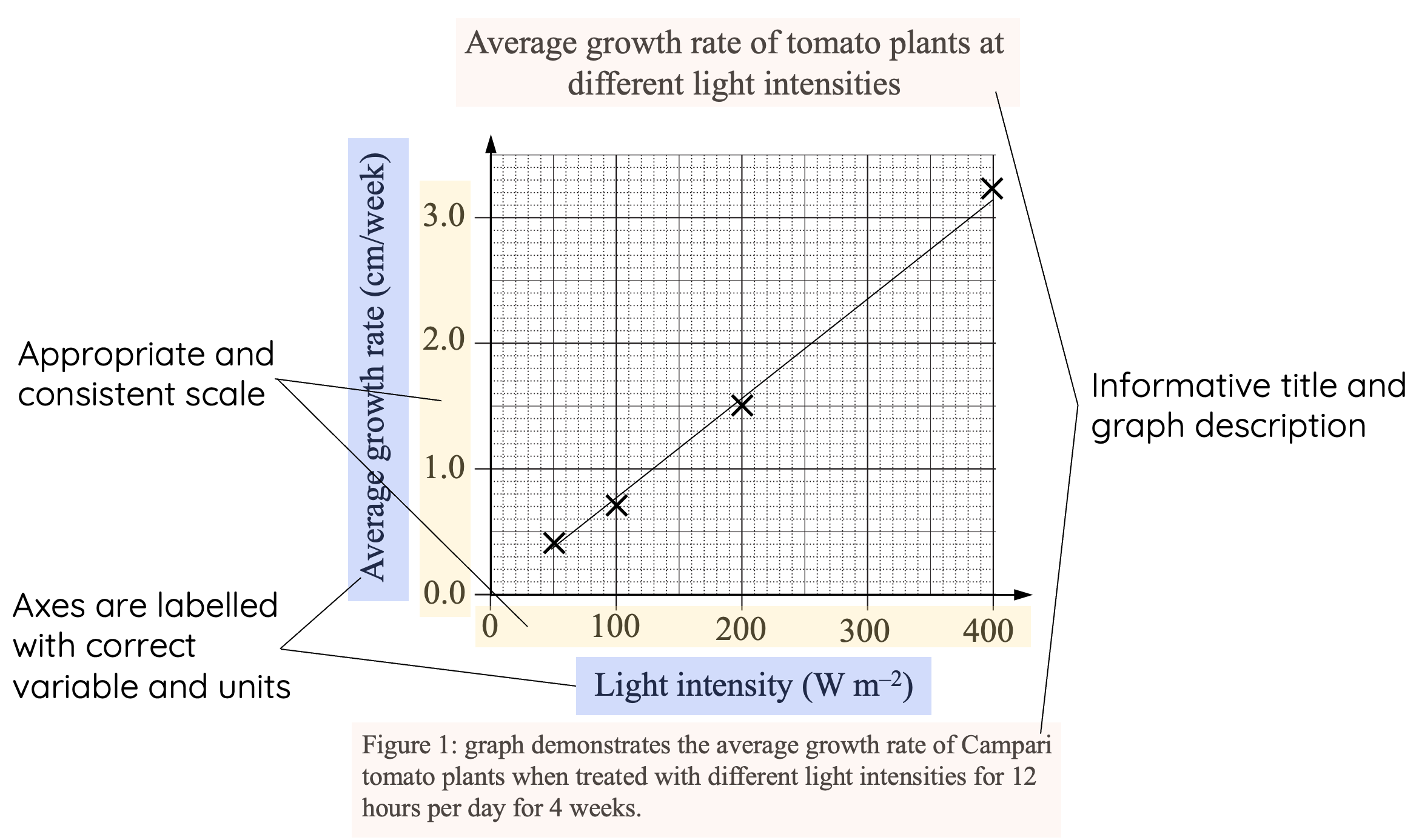# Tables and Graphs in Science

This is part of the NSW HSC science curriculum part of the Working Scientifically skills.

### How to Draw Tables and Graphs

In the NSW Higher School Certificate (HSC) Science curriculum, students are expected to present and analyse data effectively using tables and graphs. Creating clear, accurate, and well-organised tables and graphs is essential for communicating scientific findings and demonstrating a thorough understanding of the scientific method. In this article, we will discuss the key principles of drawing tables and graphs in HSC Science and provide guidelines for creating high-quality visual representations of data.

### Tables

Tables are useful for organising and presenting data in a structured and easy-to-read format. When creating tables, keep the following principles in mind:

• Table structure: Use a consistent layout with rows and columns, including clear headings for each column. Ensure that all units of measurement are specified in the headings only.
• Table formatting: Format the table neatly and consistently, with borders separating each row and column. Align numbers and text appropriately within each cell.
• Table caption: Provide a concise and informative caption that describes the content of the table. Place the caption above the table.
• Table numbering: Number tables sequentially (e.g., Table 1, Table 2) for easy referencing within your report or article.

Example:

Table 1: Growth Rate of Tomato Plants at Different Light Intensities

Light Intensity (W m⁻²) Average Growth Rate (cm/week)
50 0.4
100 0.7
200 1.5
400 3.2

### Graphs

Graphs are visual representations of data that help identify trends, patterns, and relationships between variables.When creating graphs, consider the following guidelines:

• Graph type: Choose the most appropriate type of graph for your data, such as line graphs for continuous data or bar graphs for categorical data.
• Axes: Clearly label the x and y axes, including the variable being measured and its unit (e.g., "Time (weeks)" or "Light Intensity (W m⁻²)"). The independent variable should be placed on the x-axis, while the dependent variable should be placed on the y-axis.
• Scales: Use consistent scales on both axes, with evenly spaced intervals that make it easy to read and interpret the data. The scale should such that your data points cover at least 75% of the graph.
• Data points: Plot data points accurately and clearly, using symbols or bars to represent each point.
• Lines and trendlines: For line graphs, connect data points with a smooth curve or straight line segments. If appropriate, include a line or curve of best-fit to highlight the overall pattern in the data.
• Legend: Include a legend if your graph contains multiple data series, using clear labels and symbols to distinguish between each series.
• Graph title: Provide a concise and informative title that describes the content of the graph.
• Graph numbering: Number graphs sequentially (e.g., Figure 1, Figure 2) for easy referencing within your report or article. This should be placed below the graph.

### Drawing Line of Best Fit (LOBF)

A line of best fit, also known as a trend line or regression line, is a straight line that best represents the data points in a scatter plot. It illustrates the overall trend or relationship between two variables.Here's a step-by-step guide on how to draw a line of best fit by hand:

1. Create a scatter plot: Plot your data points on a graph with the independent variable (usually time or a controlled variable) on the x-axis and the dependent variable (the variable you're measuring) on the y-axis.

2. Observe the trend: Look at the overall pattern or trend in the data points. Do they seem to follow an upward or downward trend, or is there no clear trend at all?

3. Estimate the line: Visualise a straight line that best represents the overall trend of the data points. The line should pass through or be close to as many data points as possible. The goal is to have roughly an equal number of data points above and below the line.

4. Minimise the residuals: The line of best fit should minimise the sum of the squared vertical distances (residuals) between the data points and the line itself. In other words, try to minimise the total "error" or deviation of the data points from the line. It's essential to strike a balance, as it's unlikely that all data points will lie exactly on the line.

5. Draw the line: Once you've estimated the position of the line that best represents the data points, use a ruler or a straight edge to draw the line on your scatter plot. Make sure the line of best fit does not extend beyond the plotted data points.

Keep in mind that drawing a line of best fit by hand is a subjective process, and the line you draw might differ slightly from someone else's. For a more accurate and objective method, you can use statistical software or a graphing calculator to perform a linear regression analysis, which will calculate the exact equation of the line of best fit based on your data points.